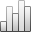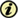Acta Stereologica

0351-580XMore Statisticssince 05 December 2013 :
View(s): 202 (1 ULiège)
Manuel Amaral Fortes

### Analysis of polar plots formed by circles or spheres

(Volume 9 (1990) — Number 1 - Proceedings of the fifth European congress for stereology - Part three - June 1990)
Article#### Abstract

Polar plots formed by circles or spheres appear in different contexts, such as in the stereology of oriented structures and in simple calculations of the surface energy of crystals. They represent functions, f(n), of the orientation, n, of a particular type, when f is proportional to the sum of the normal projections onto n of a set of given vectors, {v}. New graphical methods of analysis of these f-polar plots are developed with which the {v} set can be determined. The methods are based on the concept of principal vectors which can be determined graphically from the f-plot and from which, by another graphical method, the v-vectors are obtained as the edges of a convex polygon (2D) or polyhedron (3D). The method can also be applied to continuous distributions of v-vectors.

Three-dimensional f-plots are usually displayed in planar sections. The information on the v-vectors that can be derived from these sections is discussed. Cusps in f-plots are analysed and it is shown how their sharpness can be related to the principal vectors.

Keywords : cusps, oriented distributions, polar plots, polyhedra, rose of directions